Publicité

# Web app development_php_06

9 Jul 2013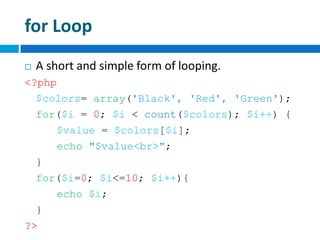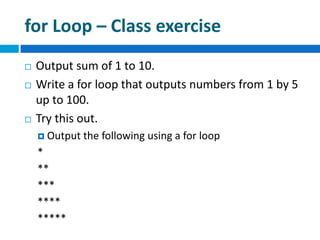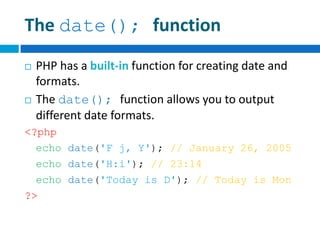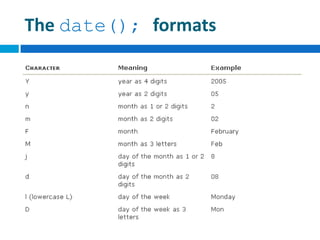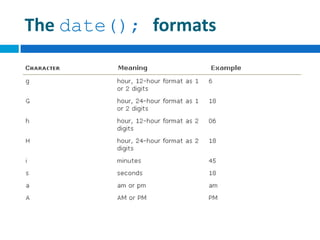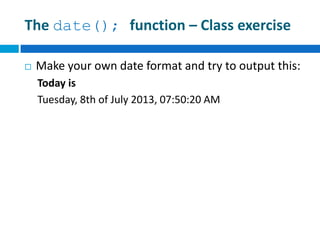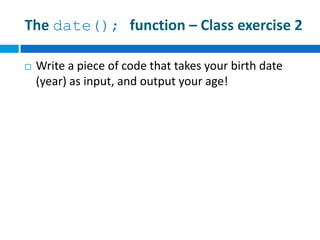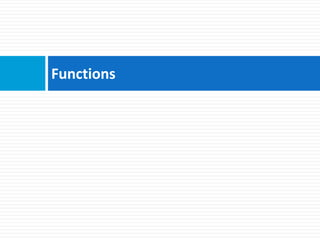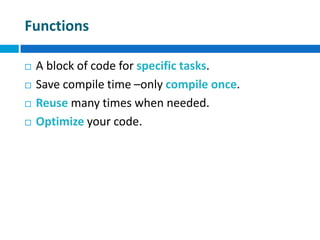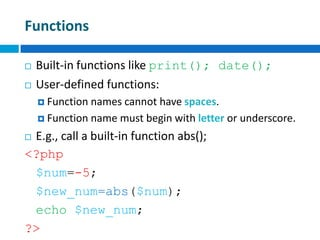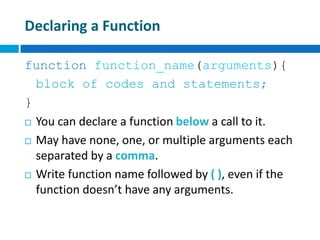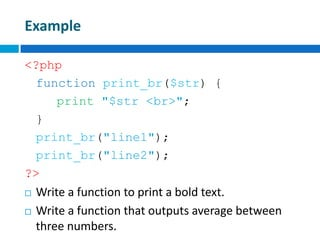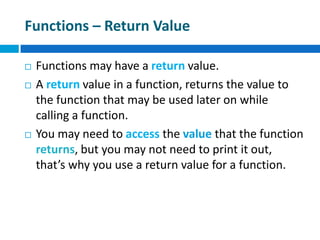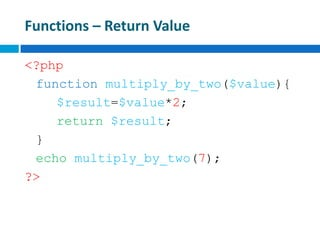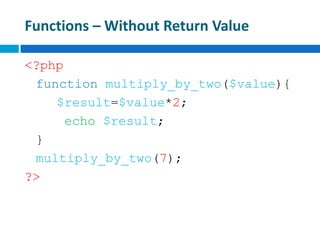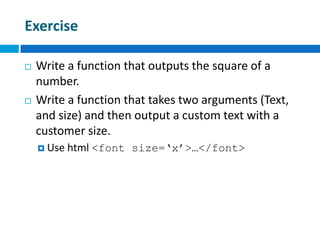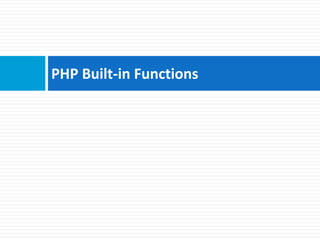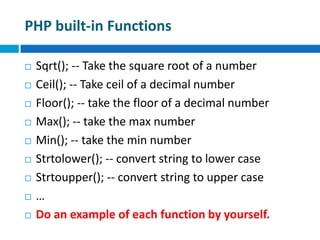1 sur 20
Publicité

### Web app development_php_06

1. hasen@microcis.net July 09, 2013Hassen poreya Trainer, Cresco Solution Afghanistan Workforce Development Program PHP Loops, Functions in PHP
2. for Loop  A short and simple form of looping. <?php \$colors= array('Black', 'Red', 'Green'); for(\$i = 0; \$i < count(\$colors); \$i++) { \$value = \$colors[\$i]; echo "\$value<br>"; } for(\$i=0; \$i<=10; \$i++){ echo \$i; } ?>
3. for Loop – Class exercise  Output sum of 1 to 10.  Write a for loop that outputs numbers from 1 by 5 up to 100.  Try this out.  Output the following using a for loop * ** *** **** *****
4. The date(); function  PHP has a built-in function for creating date and formats.  The date(); function allows you to output different date formats. <?php echo date('F j, Y'); // January 26, 2005 echo date('H:i'); // 23:14 echo date('Today is D'); // Today is Mon ?>
5. The date(); formats
6. The date(); formats
7. The date(); function – Class exercise  Make your own date format and try to output this: Today is Tuesday, 8th of July 2013, 07:50:20 AM
8. The date(); function – Class exercise 2  Write a piece of code that takes your birth date (year) as input, and output your age!
9. Functions
10. Functions  A block of code for specific tasks.  Save compile time –only compile once.  Reuse many times when needed.  Optimize your code.
11. Functions  Built-in functions like print(); date();  User-defined functions:  Function names cannot have spaces.  Function name must begin with letter or underscore.  E.g., call a built-in function abs(); <?php \$num=-5; \$new_num=abs(\$num); echo \$new_num; ?>
12. Declaring a Function function function_name(arguments){ block of codes and statements; }  You can declare a function below a call to it.  May have none, one, or multiple arguments each separated by a comma.  Write function name followed by ( ), even if the function doesn’t have any arguments.
13. Example <?php function print_br(\$str) { print "\$str <br>"; } print_br("line1"); print_br("line2"); ?>  Write a function to print a bold text.  Write a function that outputs average between three numbers.
14. Functions – Return Value  Functions may have a return value.  A return value in a function, returns the value to the function that may be used later on while calling a function.  You may need to access the value that the function returns, but you may not need to print it out, that’s why you use a return value for a function.
15. Functions – Return Value <?php function multiply_by_two(\$value){ \$result=\$value*2; return \$result; } echo multiply_by_two(7); ?>
16. Functions – Without Return Value <?php function multiply_by_two(\$value){ \$result=\$value*2; echo \$result; } multiply_by_two(7); ?>
17. Exercise  Write a function that outputs the square of a number.  Write a function that takes two arguments (Text, and size) and then output a custom text with a customer size.  Use html <font size=‘x’>…</font>
18. PHP Built-in Functions
19. PHP built-in Functions  Sqrt(); -- Take the square root of a number  Ceil(); -- Take ceil of a decimal number  Floor(); -- take the floor of a decimal number  Max(); -- take the max number  Min(); -- take the min number  Strtolower(); -- convert string to lower case  Strtoupper(); -- convert string to upper case  …  Do an example of each function by yourself.
20. hasen@microcis.net July 09, 2013Hassen poreya Trainer, Cresco Solution Any Questions!
Publicité